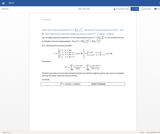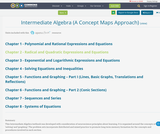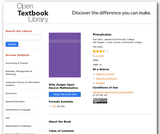Updating search results...

# 5 Results

View
Selected filters:
• Binomial TheoremConditional Remix & Share Permitted
CC BY-NC-SA
Rating
0.0 stars

College Algebra is an introductory text for a college algebra survey course. The material is presented at a level intended to prepare students for Calculus while also giving them relevant mathematical skills that can be used in other classes. The authors describe their approach as "Functions First," believing introducing functions first will help students understand new concepts more completely. Each section includes homework exercises, and the answers to most computational questions are included in the text (discussion questions are open-ended).

Subject:
Algebra
Material Type:
Textbook
Provider:
Stitz-Zeager Open Source Mathematics
Author:
Carl Stitz
Jeff Zeager
Date Added:
07/15/2011Unrestricted Use
CC BY
Rating
0.0 stars

Derivation of Euler's formula from a Taylor series expansion of the expontential function for a complex argument.Derivation of the multiple angle trig identities for sine and cosine using the Binaomial theorem and Euler's formula.

Subject:
Mathematics
Trigonometry
Material Type:
Homework/Assignment
Author:
Wissam Antypas Barakat
Date Added:
06/30/2020Unrestricted Use
CC BY
Rating
0.0 stars

Derive Euler's formula from the Taylor series of the exponential function with a complex argument.
Derive the multi-angle trigonometric identities using Euler's formula.
Derive the Binomial theorem by induction.

Subject:
Mathematics
Trigonometry
Material Type:
Homework/Assignment
Author:
Wissam Antypas Barakat
Date Added:
06/30/2020Conditional Remix & Share Permitted
CC BY-NC-SA
Rating
0.0 stars

This Intermediate Algebra textbook was developed with consideration of neuroscience principles about learning. It is organized around the concepts of 'solving' and 'graphing'. The problem sets incorporate distributed and mixed practice to promote long term memory formation for the concepts and procedures involved in each section.

Subject:
Mathematics
Material Type:
Textbook
Author:
Kathy Nabours
Shelly Dawson
Date Added:
01/19/2021Conditional Remix & Share Permitted
CC BY-NC
Rating
5.0 stars

Open source precalculus textbook.

Subject:
Calculus
Material Type:
Textbook
Provider:
Stitz-Zeager Open Source Mathematics
Author:
Carl Stitz
Jeff Zeager
Date Added:
07/15/2011